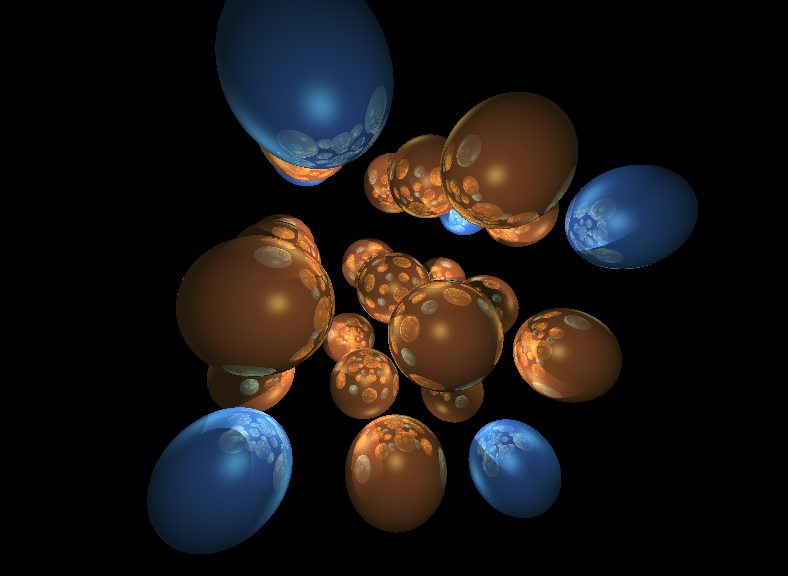# mathr / blog / #

## ray42 as a Lua package using toluaray42 was nice as it was, but I got fed up with having to recompile each time I wanted to render a new scene, and C++ isn't a particularly friendly scene description language. So I'm now using tolua to make a Lua package from the core of ray42, and it seems to work ok.

The image above was generated by this code:

require("ray")
require("materials")

local s = nil
for x = -1, 1 do for y = -1, 1 do for z = -1, 1 do
local m
if x*y*z == 0 then m = gold else m = cyan end
local t = sphere3(m, 0.5):translate(v3(x,y,z)*1.5)
if s ~= nil then s = s + t else s = t end
end end end
s = s:transform(rotate3(0.3,1,2) * rotate3(0.3,0,2) * rotate3(0.3,0,1))

local l = Lights3:new(rgb(0.25,0.25,0.25))
render3(s, l, 788, 576, 4/3, 2)
warning: deprecated conversion from string constant to 'char*'